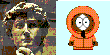David A. Kenny
November 28, 1999
PRIMER ON REGRESSION ARTIFACTS
Donald T. Campbell and David A. Kenny
Forward by Charles Reichardt

The book is about regression toward the mean:  a person who is extreme on one variable is unlikely to as extreme when measured on another variable.  I started this book with Donald T. Campbell and completed it since Don's untimely death.  Don considered regression to the mean to be one of his most important contributions in the field of methodology.  The book considers the statistical, social, biological, and political implications of regression to the mean. However, most of the focus is on quasi-experimental evaluations of over-time data.In the first chapter, we describe the phenomenon of regression toward the mean in a non-technical fashion.  We avoid presenting formulas but instead focus on a graphical presentation.  The emphasis is on the conceptual and not the mathematical.  The chapter presents the perfect correlation line (see next paragraph), the pair-link diagram, and the Galton squeeze diagram (illustrated in the paragraph), all of which are featured throughout the primer.  The Galton squeeze dramatically illustrates the more extreme scores regress to the mean.  The points on the left are the scores on a pretest, and the points on the right are the posttest means for the various scores on the pretest.  A line connects the two points.  We see that larger scores at the pretest tend to become smaller at the posttest, and smaller scores tend to become larger:  regression to the mean.This diagram is also featured in the primer.  The jagged line connected by circles is called the over-fitted line, a line in which the means are connected to form a prediction line.  The line running through the over-fitted line is the regression line or least-squares line.  The flat line is the zero-correlation line (what the regression line would be if there was no correlation) and the diagonal is the perfect correlation line (what the regression line would be if the correlation were one).  Correlation can be thought of as the relative distance of the regression line from the zero line.  The distance from the perfect correlation line to the regression line measure the amount of regression to the mean.  Regression to the mean can be viewed as far the prediction (the regression line) is from perfection (the perfect correlation line).

In the second chapter, we present the mathematics of regression toward the mean and answer commonly asked questions about regression toward the mean or FAQs.  Among the questions considered are why regression to mean does not produce mediocrity and how regression to the mean can occur when relationships are nonlinear.  We also generalize the concept beyond the simplifications of the first chapter.  Although this chapter is more mathematical than the first, we still heavily rely on graphical methods.

The next six chapters consider regression artifacts, the focus of the primer.  In Chapter 3, we show that when a group of persons are measured over time, their average score regresses toward the mean.  This chapter presents several illustrations of regression to the mean in everyday life, including the example that rookies of the year in baseball have a sophomore slump. It also considers the often ignored problem of misclassification caused by regression to the mean.  It is suggested that perhaps as many as 40% of persons classified as "extreme" (e.g., gifted, disordered, or in need of surgery) are not really extreme.

The next two chapters consider regression to the mean in the nonequivalent control group design. In this design a treated and control group are measured at two time points.  In Chapter 4, we show that matching of scores on a variable only partially controls for group differences.  Chapter 5 shows that statistical equating ("partialling out" the pretest, like matching, is not totally successful.  We argue that statistical controls are usually biased and the likely direction of bias can be determined.

Chapter 6 focuses on the measurement of change and describes regression artifacts in change score analysis.  We learn that change is a much more difficult topic than might be thought.  We show that a person who does not change at all may be the one who really "changed" the most once regression to the mean is controlled!

The next two chapters consider regression artifacts in more complicated situations. Chapter 7 considers regression to the mean in time-series research.  We focus on the problem that the timing of the intervention often occurs at an extreme point.  Chapter 8 considers longitudinal research and focuses on the idea of proximal autocorrelation.  For both of these topics we present several examples.  In Chapter 9, we review the once popular technique of cross-lagged panel correlation and urge its revival.  We show that it can be viewed as special type of multitrait-multimethod matrix.

In the final chapter, several themes are reiterated.  These themes include the utility of time-reversed analysis, graphical presentation of data, the importance of design in research, and the consideration of plausible rival hypotheses.  We also discuss how forecasters and prognosticators often fail to take into account regression toward the mean. We consider the case of Sally and Sal.  We see how Sal can be better forecaster than Sally despite the fact that Sally's predictions are much more highly correlated with the actual weather.  The chapter also considers issues of epistemology and the role of common sense in science.

Regression to the mean can be very confusing and it has confused many (including Nobel prize winners).  A goal of the book is to take much of the mystery out of this concept.